# 22 acids + bases

8 de Jun de 2016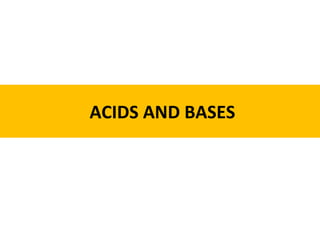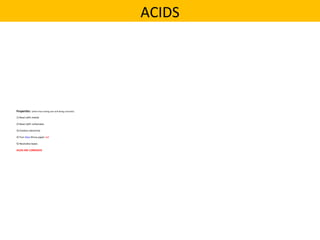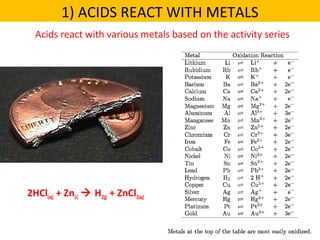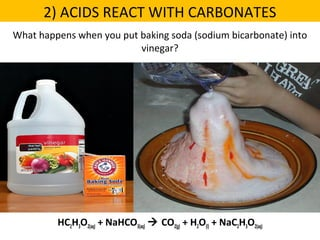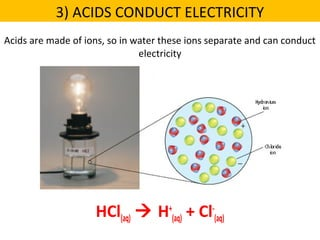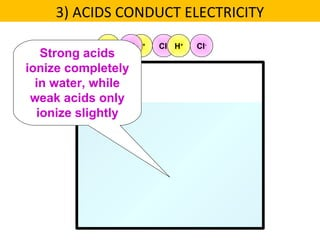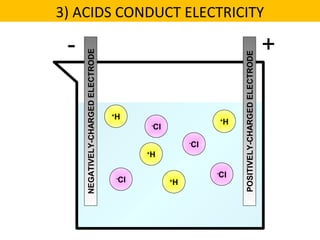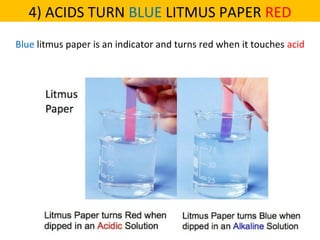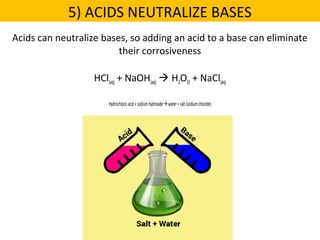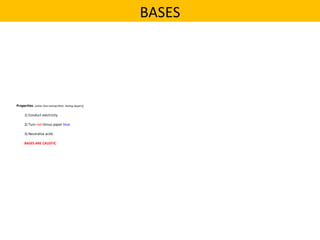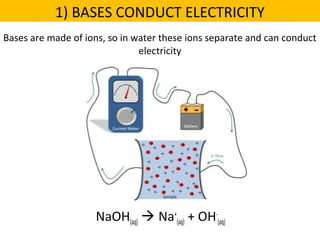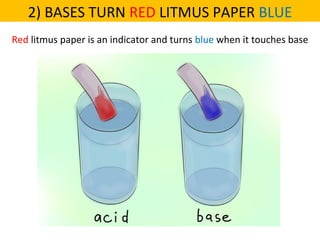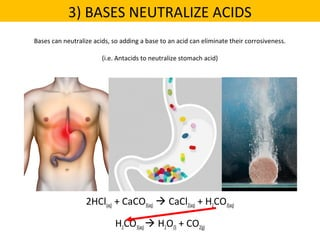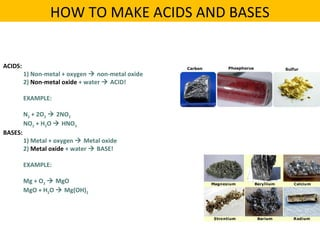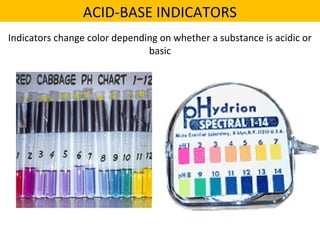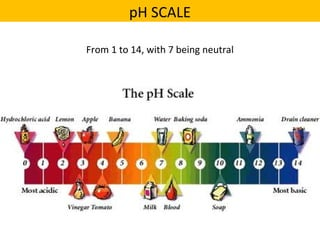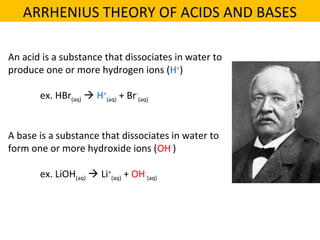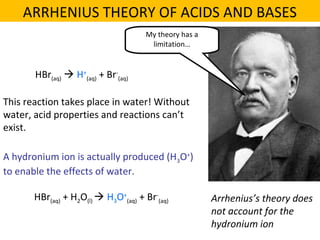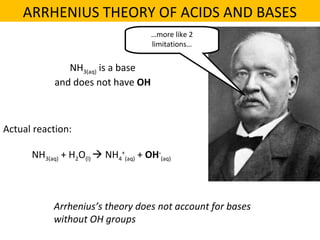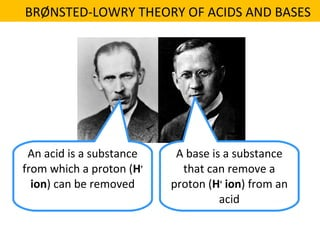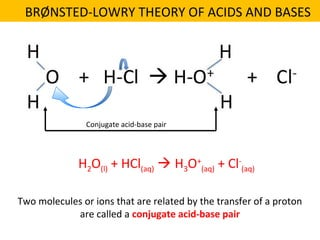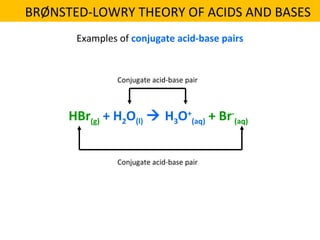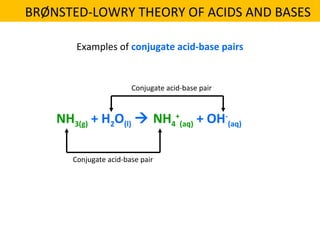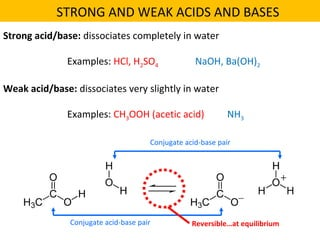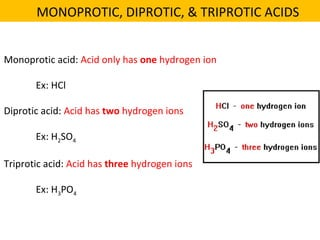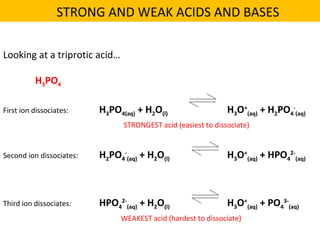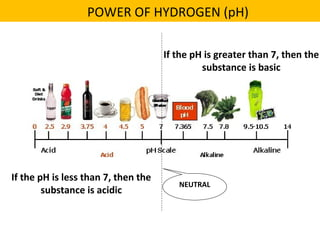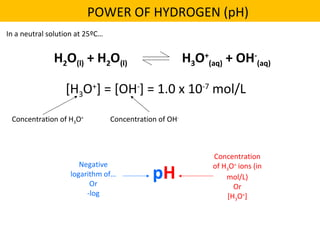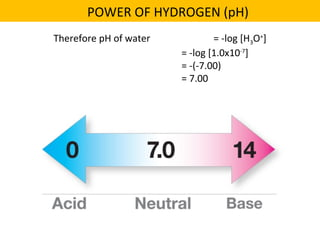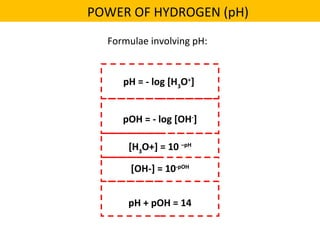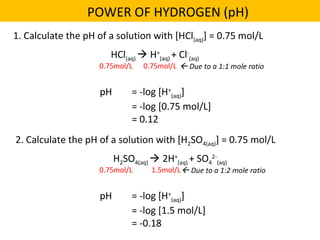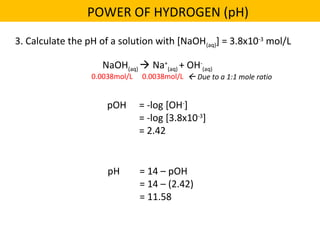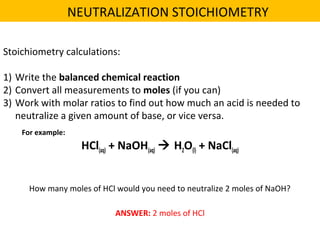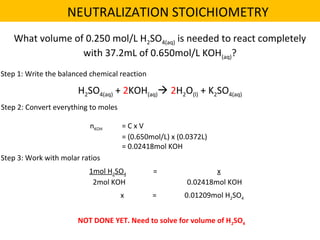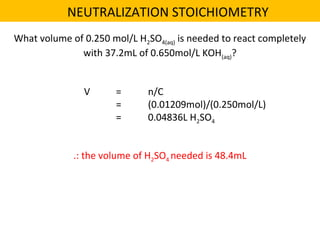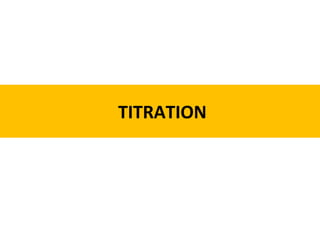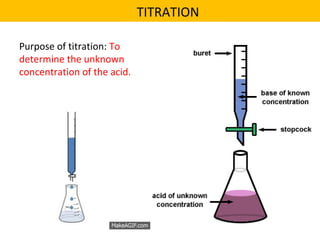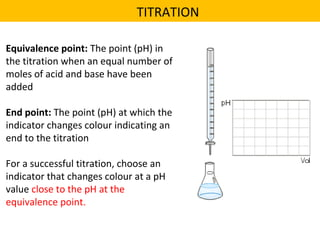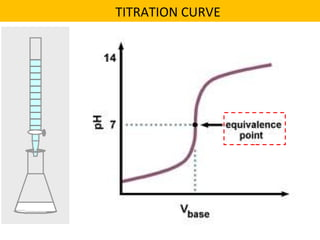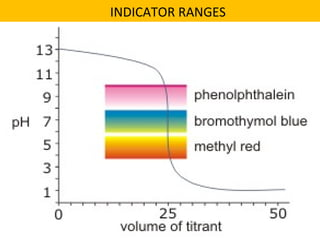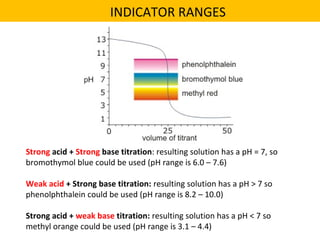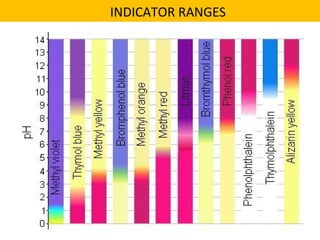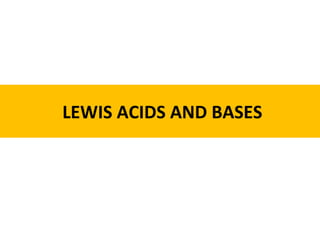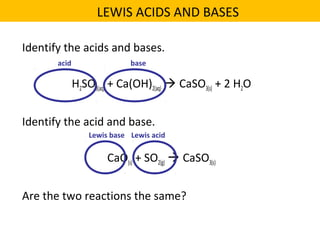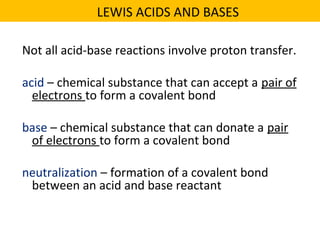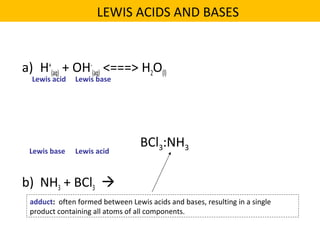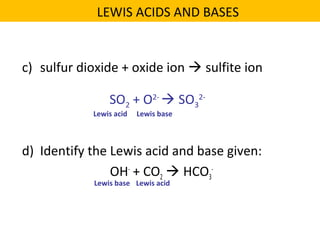1 de 47

### 22 acids + bases

• 2. Properties: (other than tasting sour and being corrosive) 1) React with metals 2) React with carbonates 3) Conduct electricity 4) Turn blue litmus paper red 5) Neutralize bases ACIDS ARE CORROSIVE ACIDS
• 3. Acids react with various metals based on the activity series 1) ACIDS REACT WITH METALS 2HCl(aq) + Zn(s)  H2(g) + ZnCl2(aq)
• 4. What happens when you put baking soda (sodium bicarbonate) into vinegar? HC2H3O2(aq) + NaHCO3(aq)  CO2(g) + H2O(l) + NaC2H3O2(aq) 2) ACIDS REACT WITH CARBONATES
• 5. Acids are made of ions, so in water these ions separate and can conduct electricity HCl(aq)  H+ (aq) + Cl- (aq) 3) ACIDS CONDUCT ELECTRICITY
• 6. H+ Cl- H+ Cl- H+ Cl- Strong acids ionize completely in water, while weak acids only ionize slightly 3) ACIDS CONDUCT ELECTRICITY
• 8. 4) ACIDS TURN BLUE LITMUS PAPER RED Blue litmus paper is an indicator and turns red when it touches acid
• 9. Acids can neutralize bases, so adding an acid to a base can eliminate their corrosiveness HCl(aq) + NaOH(aq)  H2O(l) + NaCl(aq) Hydrochloricacid+sodiumhydroxidewater+salt(sodiumchloride) 5) ACIDS NEUTRALIZE BASES
• 10. Properties: (other than tasting bitter, feeling slippery) 1) Conduct electricity 2) Turn red litmus paper blue 3) Neutralize acids BASES ARE CAUSTIC BASES
• 11. Bases are made of ions, so in water these ions separate and can conduct electricity NaOH(aq)  Na+ (aq) + OH- (aq) 1) BASES CONDUCT ELECTRICITY
• 12. Red litmus paper is an indicator and turns blue when it touches base 2) BASES TURN RED LITMUS PAPER BLUE
• 13. Bases can neutralize acids, so adding a base to an acid can eliminate their corrosiveness. (i.e. Antacids to neutralize stomach acid) 2HCl(aq) + CaCO3(aq)  CaCl2(aq) + H2CO3(aq) H2CO3(aq) H2O(l) + CO2(g) 3) BASES NEUTRALIZE ACIDS
• 14. ACIDS: 1) Non-metal + oxygen  non-metal oxide 2) Non-metal oxide + water  ACID! EXAMPLE: N2 + 2O2  2NO2 NO2 + H2O  HNO3 BASES: 1) Metal + oxygen  Metal oxide 2) Metal oxide + water  BASE! EXAMPLE: Mg + O2  MgO MgO + H2O  Mg(OH)2 HOW TO MAKE ACIDS AND BASES
• 15. Indicators change color depending on whether a substance is acidic or basic ACID-BASE INDICATORS
• 16. From 1 to 14, with 7 being neutral pH SCALE
• 17. An acid is a substance that dissociates in water to produce one or more hydrogen ions (H+ ) ex. HBr(aq)  H+ (aq) + Br- (aq) A base is a substance that dissociates in water to form one or more hydroxide ions (OH- ) ex. LiOH(aq)  Li+ (aq) + OH- (aq) ARRHENIUS THEORY OF ACIDS AND BASES
• 18. My theory has a limitation… HBr(aq)  H+ (aq) + Br- (aq) This reaction takes place in water! Without water, acid properties and reactions can’t exist. A hydronium ion is actually produced (H3O+ ) to enable the effects of water. HBr(aq) + H2O(l)  H3O+ (aq) + Br- (aq) Arrhenius’s theory does not account for the hydronium ion ARRHENIUS THEORY OF ACIDS AND BASES
• 19. …more like 2 limitations… NH3(aq) is a base and does not have OH Actual reaction: NH3(aq) + H2O(l)  NH4 + (aq) + OH- (aq) Arrhenius’s theory does not account for bases without OH groups ARRHENIUS THEORY OF ACIDS AND BASES
• 20. An acid is a substance from which a proton (H+ ion) can be removed A base is a substance that can remove a proton (H+ ion) from an acid BRØNSTED-LOWRY THEORY OF ACIDS AND BASES
• 21. H2O(l) + HCl(aq)  H3O+ (aq) + Cl- (aq) Two molecules or ions that are related by the transfer of a proton are called a conjugate acid-base pair Conjugate acid-base pair BRØNSTED-LOWRY THEORY OF ACIDS AND BASES
• 22. HBr(g) + H2O(l)  H3O+ (aq) + Br- (aq) Examples of conjugate acid-base pairs Conjugate acid-base pair Conjugate acid-base pair BRØNSTED-LOWRY THEORY OF ACIDS AND BASES
• 23. NH3(g) + H2O(l)  NH4 + (aq) + OH- (aq) Examples of conjugate acid-base pairs Conjugate acid-base pair Conjugate acid-base pair BRØNSTED-LOWRY THEORY OF ACIDS AND BASES
• 24. Strong acid/base: dissociates completely in water Examples: HCl, H2SO4 NaOH, Ba(OH)2 Weak acid/base: dissociates very slightly in water Examples: CH3OOH (acetic acid) NH3 Conjugate acid-base pair Conjugate acid-base pair Reversible…at equilibrium STRONG AND WEAK ACIDS AND BASES
• 25. Monoprotic acid: Acid only has one hydrogen ion Ex: HCl Diprotic acid: Acid has two hydrogen ions Ex: H2SO4 Triprotic acid: Acid has three hydrogen ions Ex: H3PO4 MONOPROTIC, DIPROTIC, & TRIPROTIC ACIDS
• 26. Looking at a triprotic acid… H3PO4 First ion dissociates: H3PO4(aq) + H2O(l) H3O+ (aq) + H2PO4 - (aq) Second ion dissociates: H2PO4 - (aq) + H2O(l) H3O+ (aq) + HPO4 2- (aq) Third ion dissociates: HPO4 2- (aq) + H2O(l) H3O+ (aq) + PO4 3- (aq) STRONGEST acid (easiest to dissociate) WEAKEST acid (hardest to dissociate) STRONG AND WEAK ACIDS AND BASES
• 27. If the pH is greater than 7, then the substance is basic If the pH is less than 7, then the substance is acidic NEUTRAL POWER OF HYDROGEN (pH)
• 28. H2O(l) + H2O(l) H3O+ (aq) + OH- (aq) [H3O+ ] = [OH- ] = 1.0 x 10-7 mol/L In a neutral solution at 25ºC… Concentration of H3O+ Concentration of OH- pH Negative logarithm of… Or -log Concentration of H3O+ ions (in mol/L) Or [H3O+ ] POWER OF HYDROGEN (pH)
• 29. Therefore pH of water = -log [H3O+ ] = -log [1.0x10-7 ] = -(-7.00) = 7.00 POWER OF HYDROGEN (pH)
• 30. pH = - log [H3 O+ ] pOH = - log [OH- ] [H3 O+] = 10 –pH [OH-] = 10-pOH pH + pOH = 14 Formulae involving pH: POWER OF HYDROGEN (pH)
• 31. 1. Calculate the pH of a solution with [HCl(aq)] = 0.75 mol/L POWER OF HYDROGEN (pH) HCl(aq)  H+ (aq) + Cl- (aq) 0.75mol/L 0.75mol/L  Due to a 1:1 mole ratio pH = -log [H+ (aq)] = -log [0.75 mol/L] = 0.12 2. Calculate the pH of a solution with [H2SO4(aq)] = 0.75 mol/L H2SO4(aq)  2H+ (aq) + SO4 2- (aq) 0.75mol/L 1.5mol/L Due to a 1:2 mole ratio pH = -log [H+ (aq)] = -log [1.5 mol/L] = -0.18
• 32. pH = 14 – pOH = 14 – (2.42) = 11.58 3. Calculate the pH of a solution with [NaOH(aq)] = 3.8x10-3 mol/L POWER OF HYDROGEN (pH) NaOH(aq)  Na+ (aq) + OH- (aq) 0.0038mol/L 0.0038mol/L  Due to a 1:1 mole ratio pOH = -log [OH- ] = -log [3.8x10-3 ] = 2.42
• 33. Stoichiometry calculations: 1) Write the balanced chemical reaction 2) Convert all measurements to moles (if you can) 3) Work with molar ratios to find out how much an acid is needed to neutralize a given amount of base, or vice versa. HCl(aq) + NaOH(aq)  H2O(l) + NaCl(aq) For example: How many moles of HCl would you need to neutralize 2 moles of NaOH? ANSWER: 2 moles of HCl NEUTRALIZATION STOICHIOMETRY
• 34. What volume of 0.250 mol/L H2SO4(aq) is needed to react completely with 37.2mL of 0.650mol/L KOH(aq)? H2SO4(aq) + 2KOH(aq) 2H2O(l) + K2SO4(aq) Step 1: Write the balanced chemical reaction Step 2: Convert everything to moles nKOH = C x V = (0.650mol/L) x (0.0372L) = 0.02418mol KOH Step 3: Work with molar ratios 1mol H2SO4 = x 2mol KOH 0.02418mol KOH x = 0.01209mol H2SO4 NOT DONE YET. Need to solve for volume of H2SO4 NEUTRALIZATION STOICHIOMETRY
• 35. V = n/C = (0.01209mol)/(0.250mol/L) = 0.04836L H2SO4 .: the volume of H2SO4 needed is 48.4mL NEUTRALIZATION STOICHIOMETRY What volume of 0.250 mol/L H2SO4(aq) is needed to react completely with 37.2mL of 0.650mol/L KOH(aq)?
• 37. TITRATION Purpose of titration: To determine the unknown concentration of the acid.
• 38. Equivalence point: The point (pH) in the titration when an equal number of moles of acid and base have been added End point: The point (pH) at which the indicator changes colour indicating an end to the titration For a successful titration, choose an indicator that changes colour at a pH value close to the pH at the equivalence point. TITRATION
• 41. Strong acid + Strong base titration: resulting solution has a pH = 7, so bromothymol blue could be used (pH range is 6.0 – 7.6) Weak acid + Strong base titration: resulting solution has a pH > 7 so phenolphthalein could be used (pH range is 8.2 – 10.0) Strong acid + weak base titration: resulting solution has a pH < 7 so methyl orange could be used (pH range is 3.1 – 4.4) INDICATOR RANGES
• 44. Identify the acids and bases. H2SO3(aq) + Ca(OH)2(aq)  CaSO3(s) + 2 H2O Identify the acid and base. CaO(s) + SO2(g)  CaSO3(s) Are the two reactions the same? acid base Lewis acidLewis base LEWIS ACIDS AND BASES
• 45. Not all acid-base reactions involve proton transfer. acid – chemical substance that can accept a pair of electrons to form a covalent bond base – chemical substance that can donate a pair of electrons to form a covalent bond neutralization – formation of a covalent bond between an acid and base reactant LEWIS ACIDS AND BASES
• 46. a) H+ (aq) + OH- (aq) <===> H2O(l) b) NH3 + BCl3  Lewis acid Lewis base Lewis acidLewis base BCl3:NH3 adduct: often formed between Lewis acids and bases, resulting in a single product containing all atoms of all components. LEWIS ACIDS AND BASES
• 47. c) sulfur dioxide + oxide ion  sulfite ion d) Identify the Lewis acid and base given: OH- + CO2  HCO3 - SO2 + O2-  SO3 2- Lewis acid Lewis base Lewis acidLewis base LEWIS ACIDS AND BASES